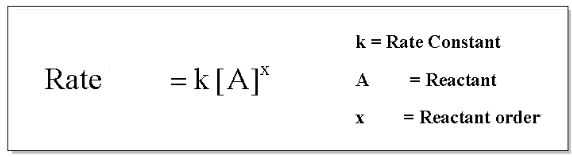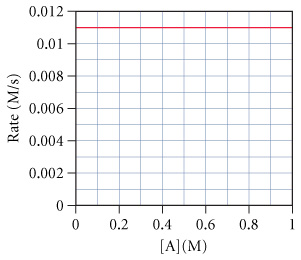# Problem: The graph below shows a plot of the rate of a reaction versus the concentration of the reactant.Estimate the value of k.

###### FREE Expert Solution

The general form of the rate law for  a reaction with a single reactant is shown below:As we can see in the equation, the rate is dependent on K and [A].

81% (43 ratings)###### Problem Details

The graph below shows a plot of the rate of a reaction versus the concentration of the reactant.

Estimate the value of k.